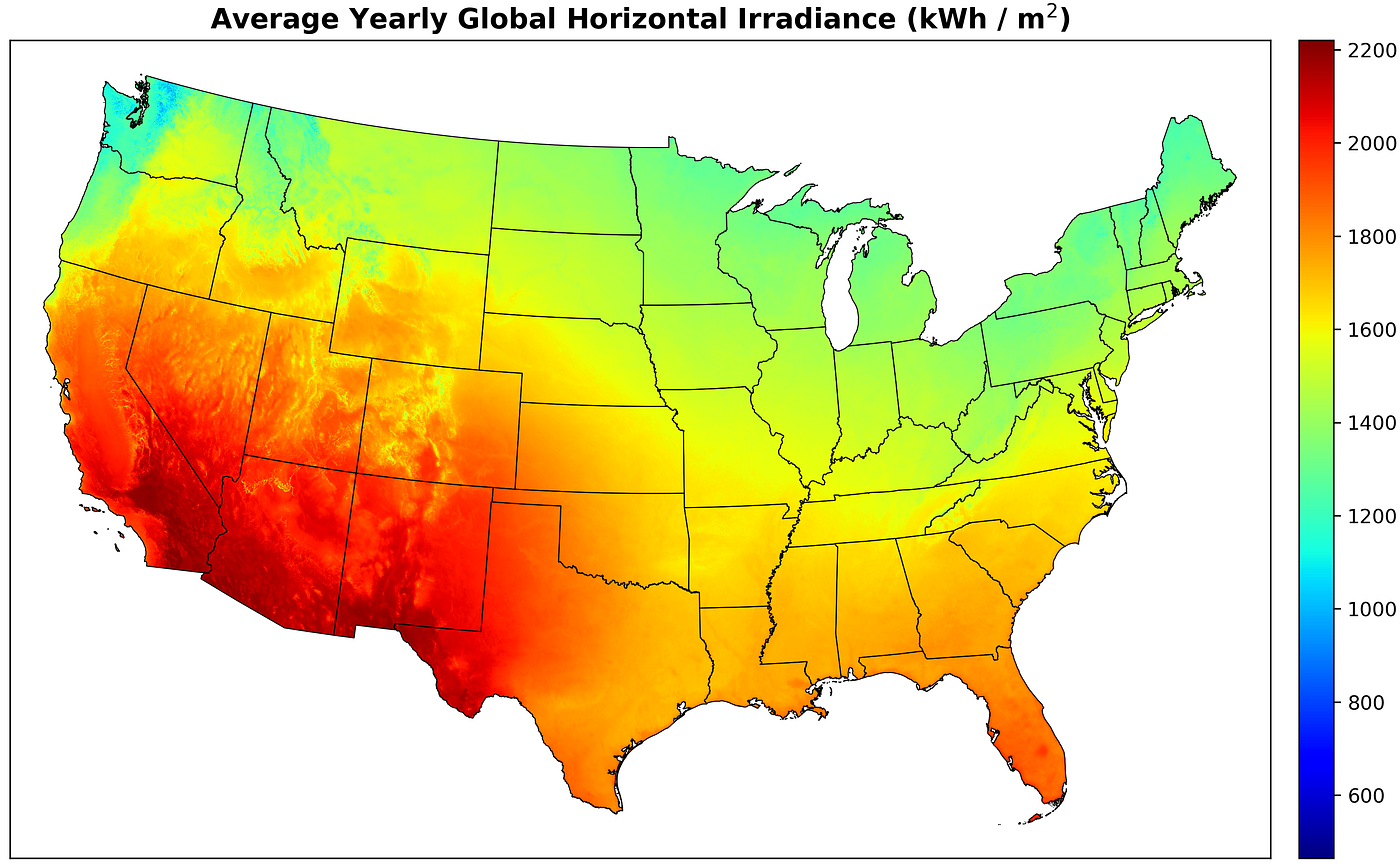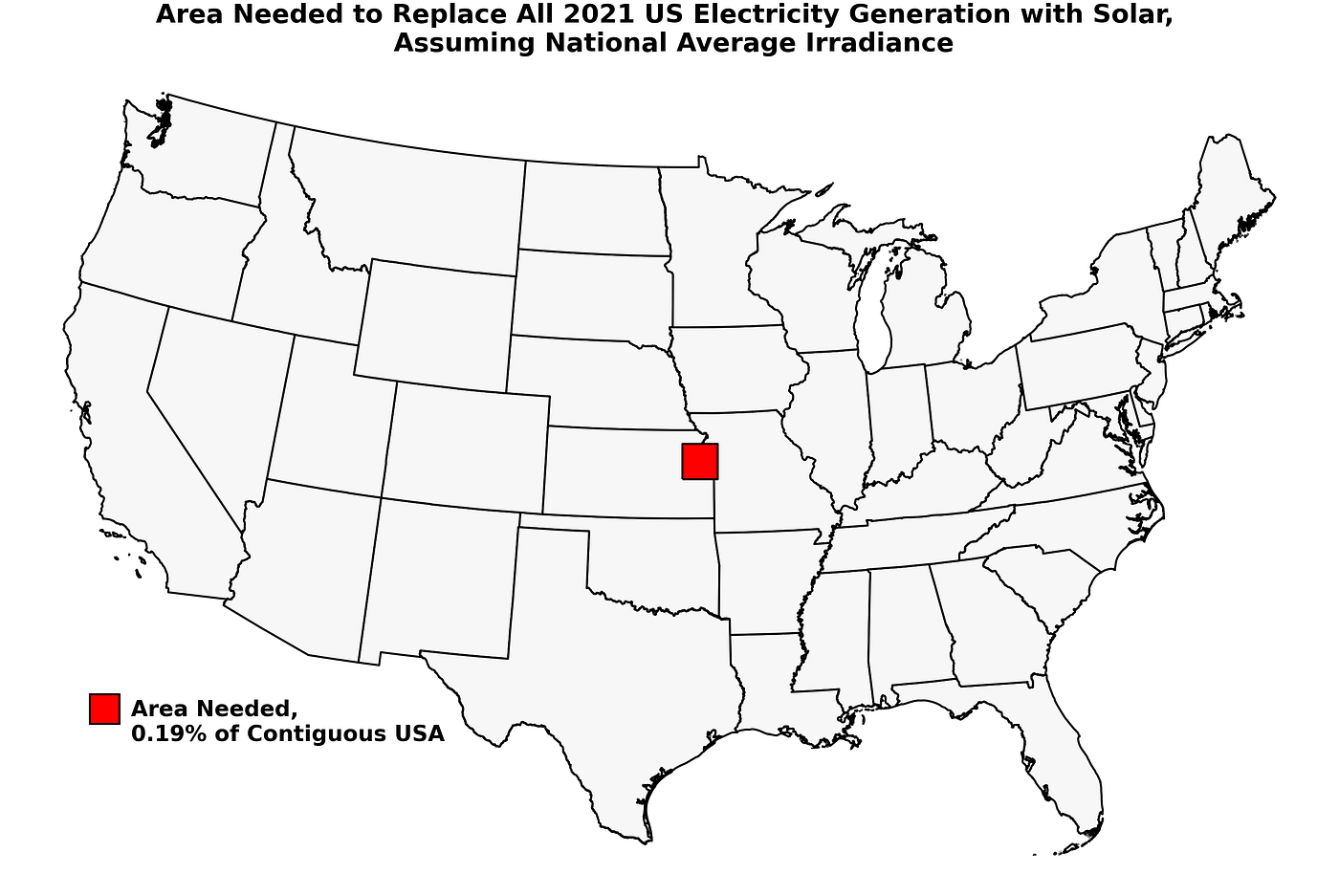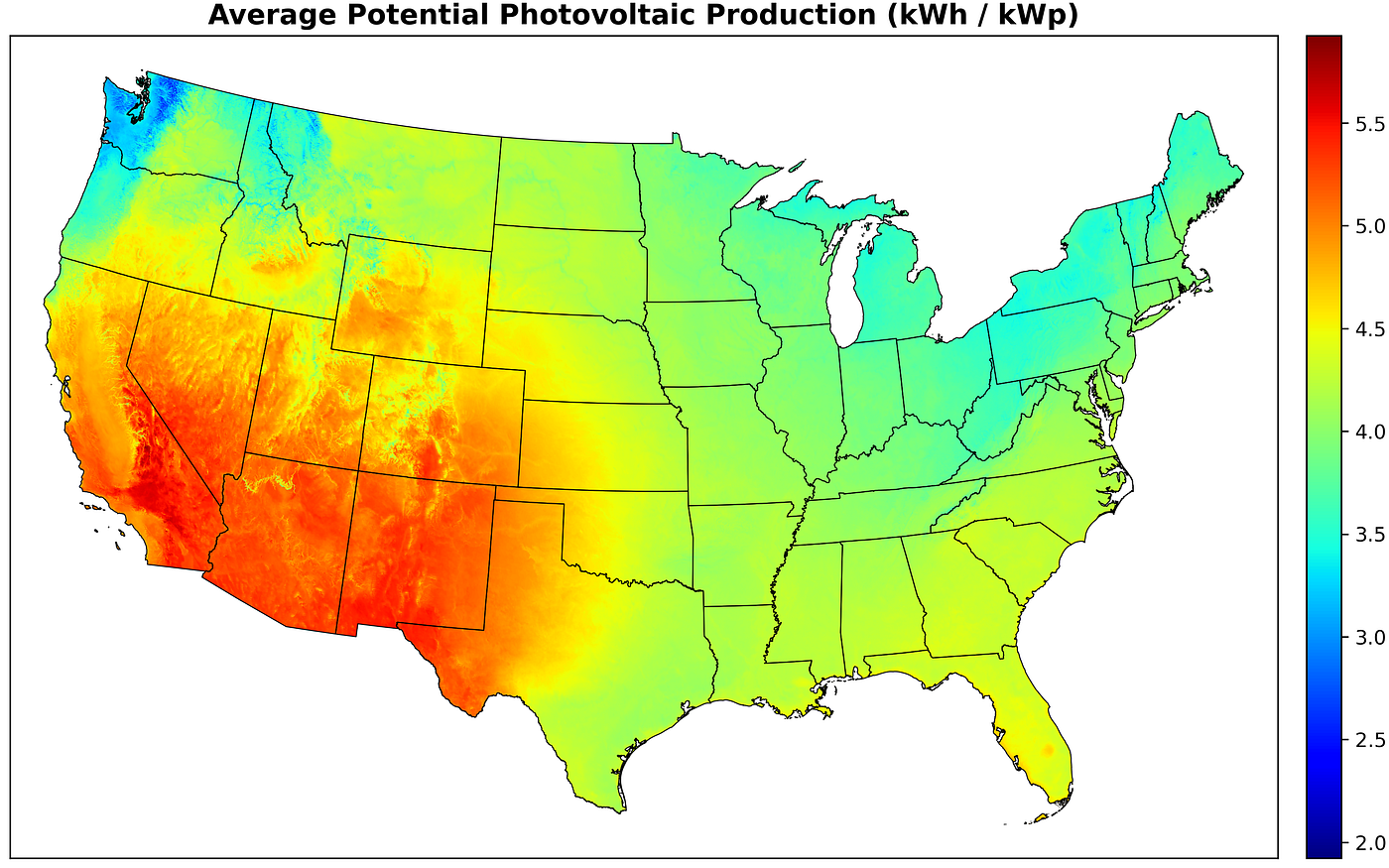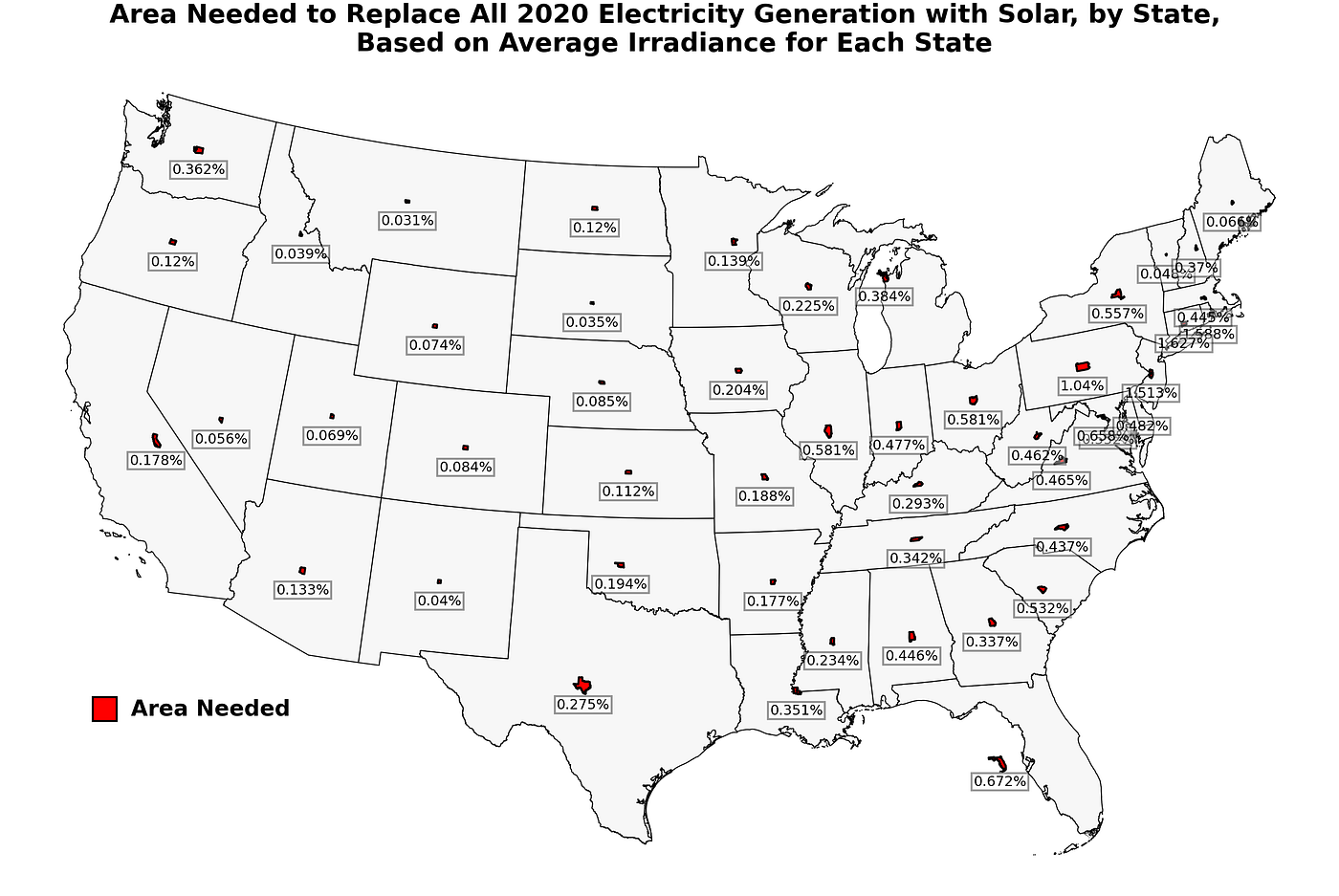# How Much Land Would it Take to Generate All US Electricity With Solar Alone?

Renewable energy resources are more diffuse than concentrated fossil energy, requiring wind farms spread o’er many leagues, and array upon array of solar panels. But is this a fundamental barrier to employing renewables at scale, as some would have you believe? The answer is nay, and it can be quickly seen from some back-of-the-envelope calculations (and with the assistance of some pretty maps), that no more than 7,000 square miles of photovoltaic panel surface area would be needed to generate 100% of US electricity. This is less than 0.2% of the contiguous US land area, and a small fraction of urban area in the US.

This demand could be satisfied with existing rooftops and other impermeable surfaces, such as parking lots. And of course, no all-renewable portfolio would actually be 100% solar, but this exercise shows that land area is not a limiting factor in scaling solar.

To inform our calculations, let us first introduce some fundamentals concerning solar resources…

# Solar Resource Fundamentals and Basic Calculation

Solar energy striking the Earth’s surface is typically measured in kilowatt-hours (kWh) per m², and the sum of sunlight that directly strikes a patch of ground, along with diffusely scattered light, is known as global horizontal irradiance (GHI). Many organizations, including the National Renewable Energy Laboratory, make detailed maps of GHI (among other metrics) over the US and larger world. The following figure shows data made publicly available by Solargis for the World Bank, giving GHI in kWh / m² per year.Figure 1. Average yearly GHI across the contiguous US. Data obtained from the Global Solar Atlas, prepared by Solargis for the World Bank. Figure by the author.

Some areas, notably the Seattle area, and parts of the Northeast, barely get 1,000 kWh per square meter, while most of the southwest gets 2,000 kWh or more. As an overall average, a square meter in the contiguous US receives 4.56 kWh daily, or about 1,663 kWh over the year. Now, we need only realize that modern solar panels convert 15–25% of incident solar radiation to electricity, and we can easily calculate the area needed to produce a given amount of electricity (which is also measured in kWh). We will also apply a conserverative performance factor of 75% to our calculation; that is, we will assume only 75% of potential production is achieved, due to factors like shading, dust, etc.

An important caveat is that global horizontal irradiance is the horizontal irradiance. That is, panels at a tilt will collect more energy than panels laying flat, but our calculations (unrealistically) imply that all photovoltaics lie flat.

# Land Needed Under Average GHI and Panels Lying Flat

Returning to the national scene, the US produced 4.116 trillion kWh in 2021, per the US Energy Information Administration (EIA). Again assuming 18% panel efficiency with a 75% performance factor gives us just over 7,000 square miles of panel needed. To put this in perspective, this is only 0.19% of the land area in the contiguous US, is a relatively small fraction of the 106,000 square miles of land classified as urban by the 2010 US Census, and is well under the area of Maricopa County in Arizona. We can visualize the area needed with the following map:Figure 2. Visual representation of the approximate land area need to replace, in principle, all 2021 Us electricity generation using solar alone. Approximately 7,000 square miles of horizontal panel would be needed, assuming 18% panel efficiency, a 75% performance factor, and US average GHI. Figure by the author.

Could this land requirement be met by rooftops alone? The answer is likely yes, as about 20–25% of urban area is actually rooftop. Using 106,000 urban square miles gives us 21,200–26,500 square miles of rooftop to work with, around 3–4 times what we need.

We can also see that this holds at a residential scale: the average residence in the US uses about 11,000 kWh of electricity per year. Under the same assumptions as above, it would take 49 m² of panel, or about 29 panels (assuming panel dimensions 65.9" by 39.25"), to meet this load. This is eminently achievable for typical single-family homes, and represents only about one-fifth of the roof area of the average new home.

# Land Needed Under Photovoltaic Potential

The calculations are conservative, and assume panels lie flat. We can get somewhat better estimates by using a map of photovoltaic potential production measured in terms of kWh per kWp: This metric includes to effect of placing panels at an optimal tilt, as well as the influence of air temperature (higher temperatures decrease solar output). kWp is the peak kilowatt rating of a photovoltaic system, and typical solar panels are in the 180–230 peak W per m² range.

We can again use data from Solargis and the World Bank to make a pretty map of photovoltaic potential output, take the average across the US, and, assuming 210 peak W per m² (plus a 75% performance factor), estimate that we need around 6,350 square miles to replace all 2021 electricity generation, or about 10% less land compared to the previous calculation.Figure 3. Average yearly potential photovoltaic production across the contiguous US, in terms of kWh per kWp. Note that this map is very similar to that in Figure 1, but the Southwest is even more clearly the optimal region for solar, while Seattle and parts of the Pacific Northwest are especially bad. Data obtained from the Global Solar Atlas, prepared by Solargis for the World Bank. Figure by the author.

If we do a more optimistic calculation, and assume 220 peak W per m², a performance factor of 80%, and that panels are overall deployed where potential production is in the top 25%, we would need only 5,300 square miles.

# A State-by-State Calculation

Finally, let’s look at each state independently. Using data on total electric power production for each state in 2020 from the EIA, and taking the average GHI for each state (using the map above), we can determine the land area needed in each state to replace all electricity with solar. Here I use the more conservative GHI-based approach above, and arrive at the following map:Figure 4. The land area required for each state to replace all 2020 electricity generation with solar is indicated visually by the tiny state polygons in red, which are scaled appropriately. The numbers give the percentage of a state’s land area needed. Land area required is calculated using the average GHI for each state, 18% efficient horizontal panels, and a 75% performance factor. Figure by the author.

As we can see, if all electricity were generated locally by solar, it would take as little as 0.031% of land area in Montana, to as much as 1.62% of land area in Connecticut, to satisfy this demand. While not ideal for solar, the sparsely populated but vast Western states tend to require extremely little land, relatively speaking, while tiny East Coast states require relatively more. In the sunny Southwest, the relatively populous Arizona and California require 0.133% and 0.178% of land area, respectively.

# Final Caveats and Conclusion

Obviously the calculations presented here do not take seasonality in electricity demand, energy storage needs, etc. into account (but they are conservative in their assumptions on solar performance and siting). However, the purpose is to give an order of magnitude estimate on how much land area is needed to meaningfully scale solar photovoltaics to a major component of the US electrical grid, and answer whether this is likely a limiting factor. The answer is clearly no, and existing urban infrastructure is likely sufficient to support all solar installations needed for our society, if wisely used.

--

--